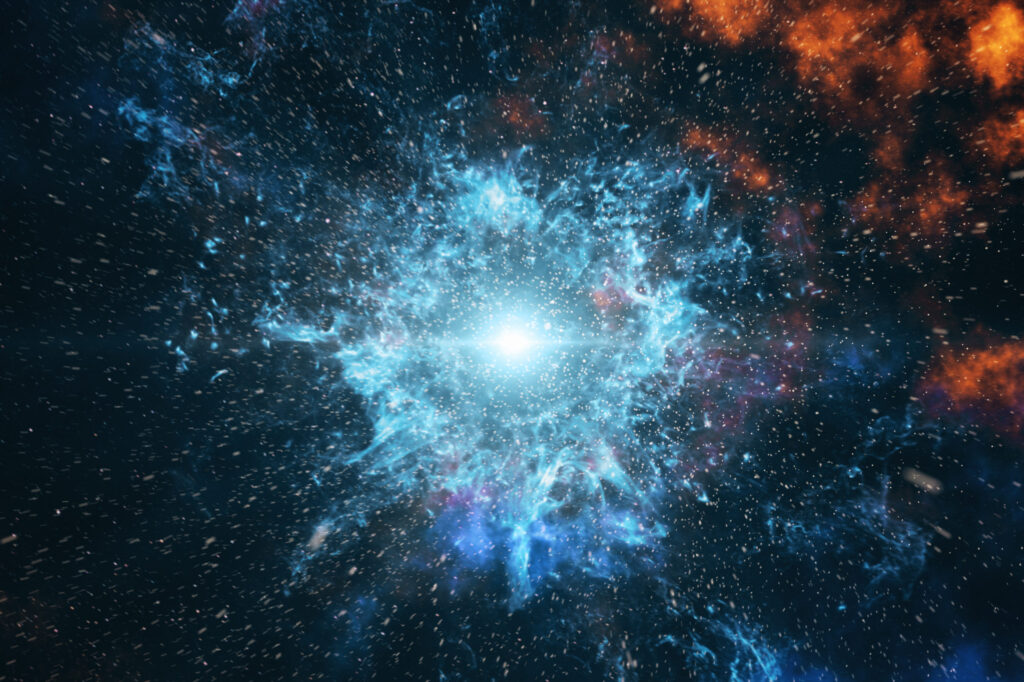# 2. Infinity Illustrates That the Universe Has a Beginning

The size of a set is how many elements it contains. The set of letters {A,B,C} and the set of girls {Shirley, Goodness, Mercy} both have a cardinality of three. In a previous post, we showed that the infinities of counting numbers and even numbers are the same.

Many subsets of the counting numbers have the same infinite size as the counting numbers. For example, consider the counting numbers and the set of numbers divisible by 10.

``            {1,2,3,4,…}``

and

``            {10,20,30,40,…}``

The size of the two sets is the same if there is a one-to-one mapping from one set to another. Here, 1 maps to 10, 2 maps to 20, 3 to 30, etc. This continues forever. The two sets are the same size.

Even the set of prime numbers has the same size as the set of counting numbers. The primes are

``            {2,3,5,7,11,13,17,…}.``

The number 5 in the set of integers maps to the 5th prime number, namely 11. The size of the sets is identical.

Things become even more curious when we are dealing with time intervals. Consider an infinite set of days

``            {day1, day2, day3,…}.``

The days go on into the future forever. A second time interval can be used. Consider

``            {week1,week2,week3,…}.``

If this set goes on, both the set of an infinite number of days and the set of an infinite number of weeks have the same infinite size. This strikes us as strange but is inarguably valid under Georg Cantor’s theory of the infinite. Day1 maps to week1, day2 to week2, and so on.

These sets can also illustrate that the universe has a beginning. The story starts with Slow Sam who is a very slow writer. Sam is writing his autobiography. But it takes Sam a week to write the account of a single day of his life. Poor Sam. He is falling further and further behind in his writing.

But in the world of Infinities this need not be the case. If the universe has always been in existence and Slow Sam has been writing for this entire infinite time, then the number of days and number of weeks today — counting from an infinite time ago — are the same. The consequence of this is crazy. If he has been writing forever, Sam can have completed his autobiography if he dies today!

This conclusion is, of course, ludicrous. Although not a definitive mathematical proof that the universe was created a finite time ago, the observation is solid evidence that our universe had a beginning. Otherwise, we would have to deal with infinity weirdnesses like Slow Sam’s autobiography.

To avoid the ridiculousness of Slow Sam finishing his autobiography, we must conclude the universe had a beginning.

Next: Reasoning with Cantor’s infinity leads to the weird conclusion that the number of points on a line segment is equal to the number of points on a square.

Here’s Part 1: Why infinity does not exist in reality. A few examples will show the absurd results that come from assuming that infinity exists in the world around us as it does in math. In a series of five posts, I explain the difference between what infinity means — and doesn’t mean — as a concept.

You may also wish to read: Yes, you can manipulate infinity in math. The hyperreals are bigger (and smaller) than your average number — and better! (Jonathan Bartlett)